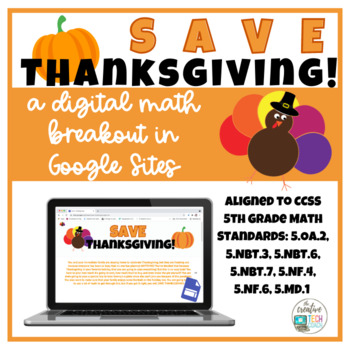DID YOU KNOW:
Seamlessly assign resources as digital activities

Learn how in 5 minutes with a tutorial resource. Try it Now

Learn More# Save Thanksgiving 2020! A Digital Math Breakout in Google SitesGrade Levels
4th - 6th
Subjects
Standards
Resource Type
Formats Included
• PDF
• Internet Activities
Compatible with Digital Devices
The Teacher-Author has indicated that this resource can be used for device-based learning.

### Description

Digital breakouts in Google Sites are an amazing way to gamify your classroom, get students engaged in content, and have them apply their knowledge in a variety of ways. Students solve clues to get codes to enter on a Google Form to save the day, solve a problem, or just get the surprise inside!

In this digital math breakout, students must solve a series of problems to save Thanksgiving! A number of skills are addressed, including adding, subtracting, multiplying, and dividing decimals; multiplying fractions; conversions of liquid measurement; finding the area of a rectangle; and interpreting numerical expressions. While this digital math breakout is aligned with 5th grade CCSS, it can be used as a challenge for younger students or review for older students, as well.

Total Pages
Answer Key
Included
Teaching Duration
N/A
Report this Resource to TpT
Reported resources will be reviewed by our team. Report this resource to let us know if this resource violates TpT’s content guidelines.

### Standards

to see state-specific standards (only available in the US).
Solve real world problems involving multiplication of fractions and mixed numbers, e.g., by using visual fraction models or equations to represent the problem.
Find the area of a rectangle with fractional side lengths by tiling it with unit squares of the appropriate unit fraction side lengths, and show that the area is the same as would be found by multiplying the side lengths. Multiply fractional side lengths to find areas of rectangles, and represent fraction products as rectangular areas.
Write simple expressions that record calculations with numbers, and interpret numerical expressions without evaluating them. For example, express the calculation “add 8 and 7, then multiply by 2” as 2 × (8 + 7). Recognize that 3 × (18932 + 921) is three times as large as 18932 + 921, without having to calculate the indicated sum or product.
Add, subtract, multiply, and divide decimals to hundredths, using concrete models or drawings and strategies based on place value, properties of operations, and/or the relationship between addition and subtraction; relate the strategy to a written method and explain the reasoning used.
Find whole-number quotients of whole numbers with up to four-digit dividends and two-digit divisors, using strategies based on place value, the properties of operations, and/or the relationship between multiplication and division. Illustrate and explain the calculation by using equations, rectangular arrays, and/or area models.

### Questions & Answers

Teachers Pay Teachers is an online marketplace where teachers buy and sell original educational materials.

More About Us